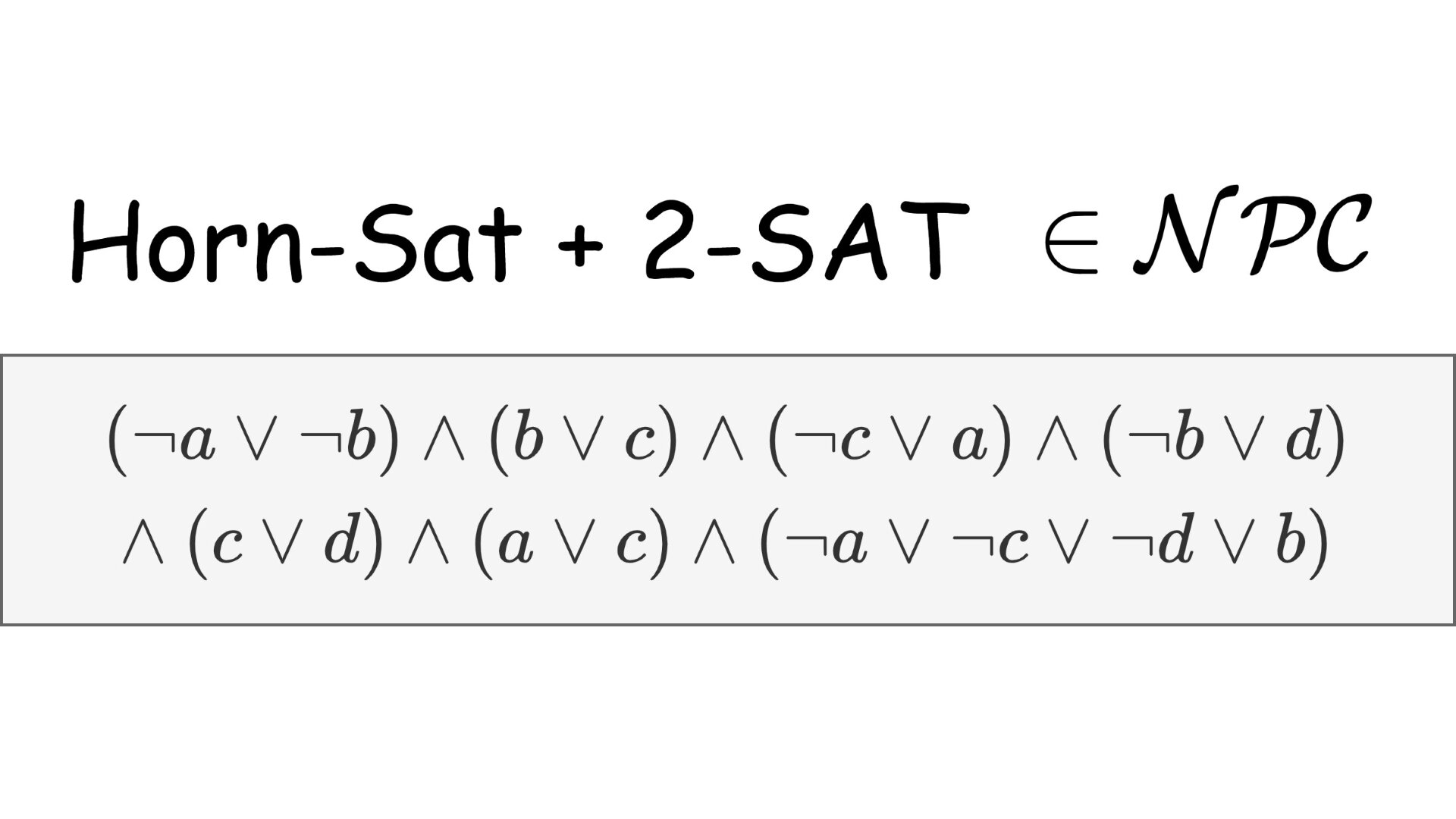# Satisfiability of formulas with both Horn and 2-SAT clauses is NP-CompleteThe SAT problem is the first problem proven to be $\mathcal{NP}$-Complete. However, many instances of this problem with certain properties can be solved efficiently in polynomial time. Well-known examples of such easy instances are 2-sat and horn formulas. All clauses in a 2-sat formula have at most 2 literals and horn-formulas consist only of clauses that contain at most one positive literal. In other words, a 2-sat clause is a conjunction of two implications and any horn clause can be rewritten as a conjunction of positive literals that implies either false or a negative literal. It may seem that satisfiability of formulas containing both 2-sat as well as horn clauses can be decided in polynomial time, however, because of the $\mathcal{NP}$-completeness that we will prove shortly, this is not the case unless $\mathcal{P} = \mathcal{NP}$. The problem also remains $\mathcal{NP}$-complete for Dual-horn clauses instead of normal horn clauses.

## The problem

The problem mentioned above can be formally formulated as follows:

Name: Horn2SAT

Input: A formula $\varphi$ in conjunctive normal form (CNF), that consists only of 2-sat or horn clauses.

Question: Is $\varphi$ satisfiable?

Example of a formula $\varphi$ that contains only 2-sat or horn clauses: $(\neg a \vee \neg b) \wedge (b \vee c) \wedge (\neg c \vee a) \wedge (\neg b \vee d) \wedge (c \vee d) \wedge (a \vee c) \wedge (\neg a \vee \neg c \vee \neg d \vee b)$

The first 6 clauses are 2-sat clauses and the last one is a horn clause, this formula is satisfiable.

## Proof of NP-Completeness

This problem is a special case of the SAT problem and is therefore also in $\mathcal{NP}$ (the variable assignment can be used as a certificate and it can be verified in polynomial time).

We will now construct a reduction from the 3-SAT problem. Consider an arbitrary 3-sat clause. If it contains at most 2 literals, that it is directly a 2-sat clause. If there is at most one positive literal, then it is already a horn clause. Therefore, we need to find equisatisfiable sets of clauses for the following situations:

1. Two positive literals: $a \vee b \vee \neg c$
2. Three positive literals: $a \vee b \vee c$

By using the idea of the Tseitin transformation for the first case, it follows that $a \vee b \vee \neg c$ is satisfiable iff $(x \leftrightarrow b \vee \neg c) \wedge (a \vee x)$ is satisfiable, this can be rewritten as: $(x \leftrightarrow b \vee \neg c) \wedge (a \vee x) \equiv \underbrace{(x \vee \neg b) \wedge (x \vee c)}_\text{2-sat clauses} \wedge \underbrace{(\neg x \vee b \vee \neg c)}_\text{Horn clause} \wedge \underbrace{(a \vee x)}_\text{2-sat clause}$

As regards the second case, we can use the result from the first case to produce a horn-clause: \begin{aligned} a \vee b \vee c &\overset{\text{sat}}{\equiv} (x \leftrightarrow a \vee b) \wedge (x \vee c) \\ &\equiv ((x \vee \neg a) \wedge (x \vee \neg b) \wedge (\neg x \vee a \vee b)) \wedge (x \vee c) \\ &\equiv (x \vee \neg a) \wedge (x \vee \neg b) \wedge (a \vee b \vee \neg x) \wedge (x \vee c) \\ &\overset{\text{sat}}{\equiv} (x \vee \neg a) \wedge (x \vee \neg b) \wedge (y \leftrightarrow b \vee \neg x) \wedge (a \vee y) \wedge (x \vee c) \\ &\equiv \underbrace{(x \vee \neg a) \wedge (x \vee \neg b) \wedge (y \vee \neg b) \wedge (y \vee x)}_\text{2-sat clauses} \wedge \underbrace{(\neg y \vee b \vee \neg x)}_\text{Horn clause} \wedge \underbrace{(a \vee y) \wedge (x \vee c)}_\text{2-sat clauses} \end{aligned}

This is clearly a polynomial-time reduction.

## Dual Horn-sat and 2-sat clauses

The above problem remains $\mathcal{NP}$-complete if we consider Dual-horn clauses instead of (normal) Horn clauses (a clause is called Dual-horn, if it has at most one negative literal).

Analogously to the proof above, in order to prove $\mathcal{NP}$-hardness we have to express 3-sat clauses with 2 and 3 negative literals in terms of 2-sat and dual-horn clauses. If the clause has 2 negative literals, then: \begin{aligned} \neg a \vee \neg b \vee c &\overset{\text{sat}}{\equiv} (\neg x \leftrightarrow \neg b \vee c) \wedge (\neg a \vee \neg x) \\ &\equiv \underbrace{(\neg x \vee b) \wedge (\neg x \vee \neg c)}_\text{2-sat clauses} \wedge \underbrace{(x \vee \neg b \vee c)}_\text{Dual horn clause} \wedge \underbrace{(\neg a \vee \neg x)}_\text{2-sat clause} \end{aligned}

Normally, in the Tseitin transformation we make the introduced variables equisatisfiable with the corresponding sub-formula. Here, in order to prevent $\neg x$ in the horn clause, we make $\neg x$ equisatisfiable with $\neg b \vee c$.

If the clause has 3 negative literals, then we use the same trick two times: \begin{aligned} \neg a \vee \neg b \vee \neg c &\overset{\text{sat}}{\equiv} (\neg x \leftrightarrow \neg a \vee \neg b) \wedge (\neg x \vee \neg c) \\ &\equiv ((\neg x \vee a) \wedge (\neg x \vee b) \wedge (x \vee \neg a \vee \neg b)) \wedge (\neg x \vee \neg c) \\ &\equiv (\neg x \vee a) \wedge (\neg x \vee b) \wedge (\neg a \vee \neg b \vee x) \wedge (\neg x \vee \neg c) \\ &\overset{\text{sat}}{\equiv} (\neg x \vee a) \wedge (\neg x \vee b) \wedge (\neg y \leftrightarrow \neg b \vee x) \wedge (\neg a \vee \neg y) \wedge (\neg x \vee \neg c) \\ &\equiv \underbrace{(\neg x \vee a) \wedge (\neg x \vee b) \wedge (\neg y \vee b) \wedge (\neg y \vee \neg x)}_\text{2-sat clauses} \wedge \underbrace{(y \vee \neg b \vee x)}_\text{Dual horn clause} \wedge \underbrace{(\neg a \vee \neg y) \wedge (\neg x \vee \neg c)}_\text{2-sat clauses} \end{aligned}